# Foxes hunting Bunnies: Population Modelling with the Predator-Prey Equations

in #steemstem2 years ago (edited)

Let's take a peek into a more advanced area of study in the field of differential equations. A few days ago @mathowl posted a fascinating article: "The mathematics behind medieval battles" and mentioned that the model is connected to the Predator-Prey equations.

So I thought we could explore this model a bit further and develop the logic behind it.

Suppose we have a prey species (for example: rabbits) that serves as a food source for a predator species (for example: foxes).Image Source

First, let the number of rabbits (i.e. population) be denoted by R.

Now, assuming there are no predators around, and they have indefinite lives, and infinite resources and space in which to thrive, the growth in the population of rabbits can be modeled by natural growth (aka exponential growth), or the equation...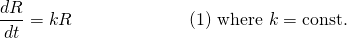For foxes, assuming they have no food supply (no prey) we would expect their population, denoted by F to naturally decline (aka exponential decay) according to the equation...Let's now bring predator and prey together so they start encountering or "interacting" with each other. So let the number of encounters be the product of the number of foxes by the number of rabbits.

Obviously this is very simplistic, but what this means is the number of encounters depends on the number of either species. So we define...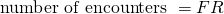Unfortunately, for the prey that means being eaten by the predators. So we would expect their population growth to be slowed in proportion to the number of encounters, expressed as. Thus for the poor bunnies...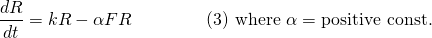For the predators, we would expect the change in their population to be boosted in proportion to the number of encounters since they now have a food source (). So for foxes, we have...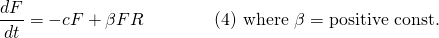Equations (3) and (4) together, are a system of first order differential equations called the "Lotka-Volterra" equations (or simply, predator-prey equations).They are a pair of non-linear differential equations which must be solved simultaneously, and the solution is likewise, a pair of functions R(t) and F(t).

Now unfortunately, explicit solutions to R(t) and F(t) are usually impossible to find. Most of the time, they are approximated by "linearising" the non-linear system, or using a numerical integrating method. This is too complex for us (i.e. for me, as I'm still revising and learning) at this stage, but we still have plenty to explore...

For the following example, let's set some values...

• k = 0.05
• c = 0.018
• α = 0.002
• β = 0.00002

## Equilibrium solutions to the Lotka-Volterra Equations

As always, equilibrium points (or critical points) are where the rate of change in population is equal to zero. To find these, we simply set equation (3) equal to 0...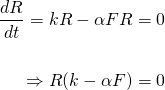The above as solutions R = 0 and F = k/α = 0.05/0.002 = 25.

Likewise, we also set equation (4) equal to 0...Has solutions F = 0 and R = c/β = 0.018/0.0002 = 900.

What this physically means is populations of either species can be sustained indefinitely when...

1. There are no foxes and rabbits in the ecosystem whatsoever, (R,F) = (0,0). And this sort of makes sense because if neither species existed in the first place, then their populations would not change (unless they may evolve into existence over a very long time ... but that's a controversial debate for another day).

2. The number of foxes is 25 and the number of rabbits is 900 (R,F) = (900,25). Or in other words, 900 rabbits is just enough to support 25 foxes. There are neither too few foxes such that the population of rabbits increases, nor too many foxes such that their numbers decline.

## Phase portrait solution

While we may not easily be able to obtain the functions R(t) and F(t), we can still obtain in my opinion, an even more meaningful relationship between the number of foxes to rabbits with what is known as a phase portrait that is independent of time.

To do this, we use the chain-rule to eliminate time t and find an expression for dF/dR...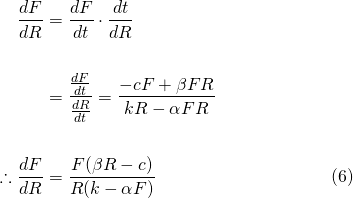The direction field shown in Figure 1 will give us an idea of the solutions we can obtain from equation (6)...Figure 1.

Equation (6) is a separable differential equation. So, the variables can be separated and then the equation solved by integrating both sides...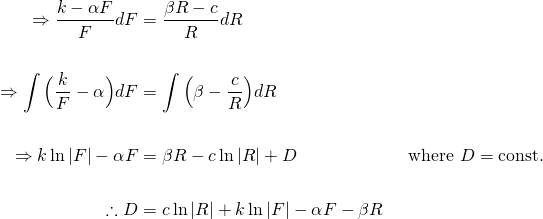Substituting the numerical values, we get the general solution...Several solution curves of the phase portrait for foxes versus rabbits is shown in Figure 2.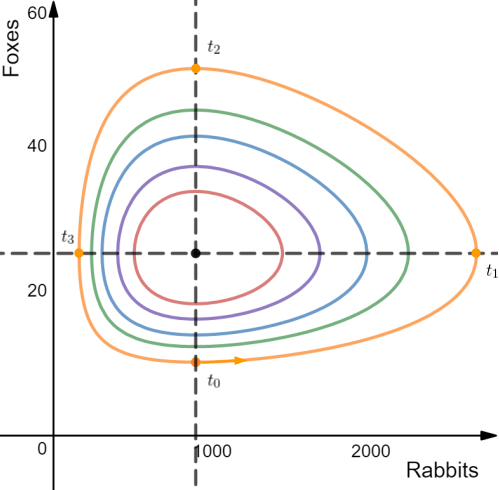Figure 2.

The egg shaped curves show how the population of either species changes with respect to each other. The equilibrium solution of 900 rabbits to 25 foxes lies at the centre of these curves.

The outer orange curve in Figure 2 is a particular solution where we start at t0 with 900 rabbits and only 10 foxes.

If we follow this curve in the anti-clockwise direction as time progresses, we see that the increase in the number of foxes is very gradual compared to the number of bunnies because there are not enough foxes to significantly slow the increase in their population.

However, because the foxes have plenty of food (prey), their rate of population increase gets faster and faster until there are about 2800 rabbits in the ecosystem, the point at which the population of rabbits begins to decrease, t1. The number of rabbits cannot continue to grow because there are 25 foxes catching them.

The fox population continues to grow as the number of rabbits decreases albeit at a slower rate until they number 50 at t2. After this, both populations are in decline. There are too many foxes and they have consumed so many rabbits that they are exhausting the food supply, and their numbers decline rapidly.

At t3, foxes are still in rapid decline while the rabbit population starts to recover and increase again. As time goes on, the rate of fox depopulation slows as their food supply increases again until we reach a point similar to when we started at t0.

A typical plot of each population over time is shown in Figure 3 below. As you can see, there is a phase lag for the predator population which makes sense according to the description of the phase portrait above.Figure 3.
Image Source

## Assumptions of the standard Predator-Prey model

The example described above is the standard model first described by Lotka & Volterra in the 1920's. Several assumption are made to simplify the model that are not necessarily realisable in nature.

They are:

1. The prey population finds ample food at all times.
2. The food supply of the predator population depends entirely on the size of the prey population.
3. The rate of change of population is proportional to its size.
4. During the process, the environment does not change in favour of one species and genetic adaptation is inconsequential.
5. Predators have limitless appetite.

Source: Wikipedia

Many variations of the Lotka-Volterra equations have been proposed to take into account natural factors (such as the environment, food supply for the prey etc.) that may effect the growth rates of the species in question. We'll leave these for another time.

Credits:

All equations in this tutorial were created with QuickLatex

All graphs were created with www.desmos.com/calculator

## First order Non-linear Differential Equations

1. There's a hole in my bucket! Let's turn it into a cool Math problem!
2. The Calculus of Hot Chocolate Pouring!
3. Foxes hunting Bunnies: Population Modelling with the Predator-Prey Equations

Please give me an Upvote and Resteem if you have found this tutorial helpful.

I would really appreciate any small donation which will help me to help more math students of the world.

Tip me some DogeCoin: A4f3URZSWDoJCkWhVttbR3RjGHRSuLpaP3

Tip me at PayPal: https://paypal.me/MasterWu

Sort:

There is a lot of literature about studying this system using geometric qualitative methods.

Absolutely @nenio. Some fascinating studies have been done with these models.

You've got our team services. [@steemsoul--@rayhanahmed--@shahanasayan--@raselhossain] Thanks for using our service.

Nice article, as always! I have a question: How is the direction field in Figure 1 derived? Differential equations aren't my strength and I haven't seen this type of graph yet.

2 years ago (edited)

If you're asking mathematically, direction fields are simply drawn by choosing values of F and R and working out dF/dR from those chosen values. dF/dR is then simply the slope at the chosen (F, R). So say when you choose F to be 20, at R = 100 the slope dF/dR is quite steep in the negative direction and gradually flattens and becomes positive for increasing values of R.

Graphically, it is drawn in Desmos using this tool: https://www.desmos.com/calculator/p7vd3cdmei

I was asking for exactly that! Thanks. So basically, there is no definite resolution to this, you could technically choose as many points as you liked and calculate the slope at that point, correct?

2 years ago (edited)

That's right. There are an infinite number of solutions. The direction field will give you an idea of what a particular solution might look like because it shows you how the slope changes from point to point.

Huh. I wonder if one could increase the resolution to say, the resolution of your monitor and color code the slopes instead of using lines to get a more detailled field. I am not sure if this is at all necessary or helpful, but it seems like a fun project nonetheless.

2 years ago (edited)

Sure. Why not? I guess it depends on your goal. For global behaviour of a differential equation, increasing the number of points and resolution may not add so much additional benefit - it may even have the opposite effect of increasing clutter.

The one I have drawn in this post is borderline in my opinion. I could have made the little slope lines a bit bigger and used 1/2 the amount of points, and the slope field (aka direction field) would have told the same story.

However, if you want to zoom in on a behaviour near a critical point, you may want to increase the resolution to accurately depict what's going on.

2 years ago (edited)

To expand on the answer a bit. So for 2-dimensional first order ODEs there is the Poincaré–Bendixson theorem. This tells you all the possible types of solutions that can occur. Using this and studying the vector field it is easy to prove which solutions occur.

In the case of equilibria (for n-dimensional ODEs) you have the Stable Unstable and Center Manifold Theorem. These theorems tell you what happens close to an equilibrium.

Nice article @masterwu and thanks for the complement :o)

Thanks @mathowl. This a fantastic platform with a brilliant STEM community. Very motivating!

A great overview of the model. What it does not take into account is the other prey of the foxes.

If there is abundant alternative prey, both the rabbit and fox population will continue to grow, as can be seen in Australia.

And it does not provide a solution to the age old question - which goes first, the rabbit or the fox? Remove rabbits and foxes switch to native animals for prey, remove foxes and rabbit populations boom. Add cats and the problem becomes a mess.

Thanks @andyfishman. There are versions of these equations which include other prey of the predators, but that makes my working a lot more complex, haha. So I'm avoiding this at this stage.

And the rest of what you say goes to show, it's impossible to solve the world's problems with mathematics alone. :)

Sneaky Ninja Attack! You have been defended with a 2.80% vote... I was summoned by @masterwu! I have done their bidding and now I will vanish...Whoosh

You got a 1.11% upvote from @allaz courtesy of @masterwu!

You got a 1.57% upvote from @minnowvotes courtesy of @masterwu!

This post has received a 2.10 % upvote, thanks to: @masterwu.

This post has received a 1.65% upvote from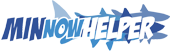thanks to: @masterwu.